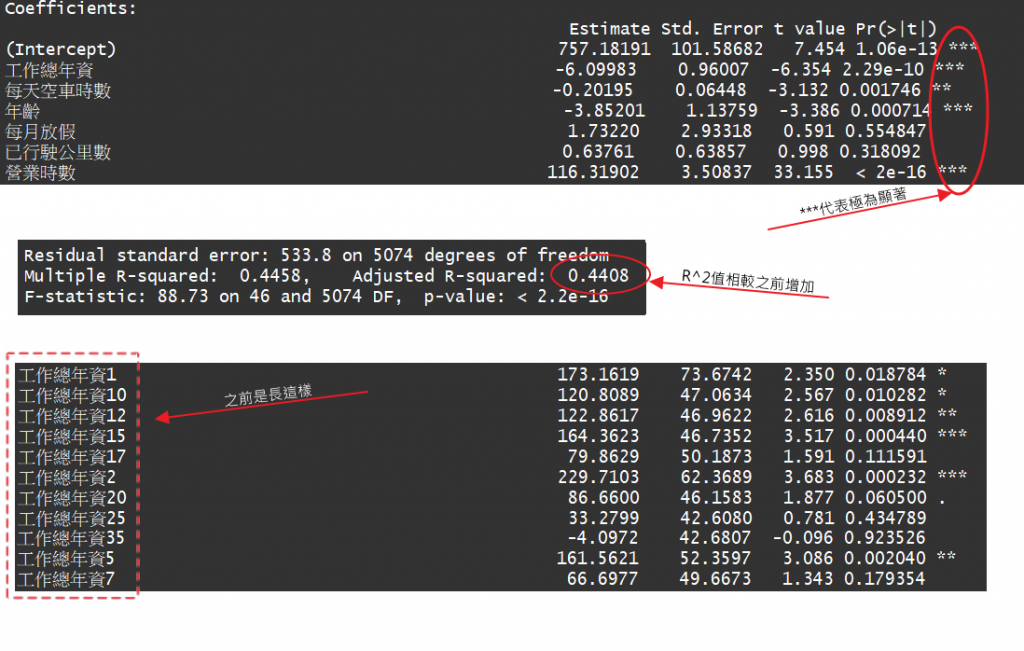#2019 iT 邦幫忙鐵人賽DAY 13
0
AI & Data

## [Day 13] 政府開放資料_計程車營運狀況調查_回歸(數值與類別變數補充)

``````xx[,"每天空車時數"] = as.numeric(as.character(xx[,"每天空車時數"]))
xx[,"年齡"] = as.numeric(as.character(xx[,"年齡"]))
xx[,"每月放假"] = as.numeric(as.character(xx[,"每月放假"]))
xx[,"已行駛公里數"] = as.numeric(as.character(xx[,"已行駛公里數"]))
xx[,"營業時數"] = as.numeric(as.character(xx[,"營業時數"]))
xx[,"工作總年資"] = as.numeric(as.character(xx[,"工作總年資"]))
``````